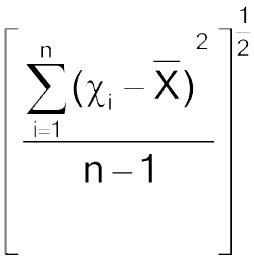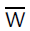# Online Calculation of Acceptance Value for Uniformity of dosage units test as per USP chapter <905> Uniformity of dosage units test

Online Calculation of Acceptance Value (av) for Uniformity of dosage units test is useful tool for pharma professionals for quickely calculate the results online without storing data on website.

The USP <905> general chapter is harmonized with the corresponding texts of the European Pharmacopoeia and the Japanese Pharmacopoeia.

## Calculation of Acceptance Value for Uniformity of dosage units test

 Unit L1 stage Insert values for L2 stage 1 2 3 4 5 6 7 8 9 10

## Content uniformity Results

 L1 stage results Total Units RSD A Value Remark L2 stage results Total Units RSD A Value Remark Input Data Value Min.Value Max.Value Max allowed range for individual unit Min.Value Max.Value

## Acceptance Value and Content Uniformity

 L1 Maximum allowed acceptance value L1 = 15.0 unless otherwise specified L2 L2 = 25.0 unless otherwise specified Maximum allowed range for deviation of each dosage unit tested from the calculated value of M. On the low side, no dosage unit result can be less than [1–(0.01)(L2)]M, while on the high side, no dosage unit result can be greater than [1 + (0.01) (L2)]M. (This is based on an L2 value of 25.0.)

The USP <905> general chapter is harmonized with the corresponding texts of the European Pharmacopoeia and the Japanese Pharmacopoeia.

To ensure the consistency of dosage units, each unit in a batch should have a drug substance content within a narrow range around the label claim. Dosage units are defined as dosage forms containing a single dose or a part of a dose of drug substance in each unit. The uniformity of dosage units specification is not intended to apply to suspensions, emulsions, or gels in unit-dose containers intended for external, cutaneous administration.

As per USP, the term “uniformity of dosage unit” is defined as the degree of uniformity in the amount of the drug substance among dosage units.

The uniformity of dosage units can be demonstrated by either of two methods, Content Uniformity or Weight Variation. The test for Content Uniformity of preparations presented in dosage units is based on the assay of the individual content of drug substance(s) in a number of dosage units to determine whether the individual content is within the limits set. The Content Uniformity method may be applied in all cases. The test for Weight Variation is applicable for the following dosage forms:

W1: Solutions enclosed in unit-dose containers and into soft capsules;

W2: Solids (including powders, granules, and sterile solids) that are packaged in single-unit containers and contain no active or inactive added substances;

W3: Solids (including sterile solids) that are packaged in single-unit containers, with or without active or inactive added substances, that have been prepared from true solutions and freeze-dried in the final containers and are labeled to indicate this method of preparation; and

W4: Hard capsules, uncoated tablets, or film-coated tablets, containing 25 mg or more of a drug substance comprising 25% or more, by weight, of the dosage unit or, in the case of hard capsules, the capsule contents, except that uniformity of other drug substances present in lesser proportions is demonstrated by meeting the requirements for Content Uniformity.

## CONTENT UNIFORMITY:

Select not fewer than 30 units, and proceed as follows for the dosage form designated.

## Solid Dosage Forms:

Assay 10 units individually using an appropriate analytical method. Calculate the acceptance value.

## Liquid or Semi-Solid Dosage Forms:

Assay 10 units individually using an appropriate analytical method. Carry out the assay on the amount of well-mixed material that is removed from an individual container in conditions of normal use, and express the results as delivered dose. Calculate the acceptance value.

## Calculation of Acceptance Value:

Calculate the acceptance value by the formula:(Mean) Mean of individual contents (χ1, χ2, …, χn), expressed as a percentage of the label claim χ1, χ2, …, χn Individual contents of the units tested, expressed as a percentage of the label claim n Sample size (number of units in a sample) k Acceptability constant If n = 10, then k =2.4 If n = 30, then k =2.0 s Sample standard deviationRSD Relative standard deviation (the sample standard deviation expressed as a percentage of the mean) 100s/ Mean of individual contents M (case 1) to be applied when T ≤101.5 Reference value: If 98.5% ≤ Mean ≤101.5%, then M = Mean (AV = ks) If Mean <98.5%, then M = 98.5% (AV = 98.5 – Mean + ks) If Mean >101.5%, then M = 101.5% (AV = Mean – 101.5 + ks) M (case 2) to be applied when T >101.5 Reference value: If 98.5 ≤Mean ≤T, then M = Mean (AV = ks) If Mean <98.5%, then M = 98.5% (AV = 98.5 – Mean + ks) If Mean >T, then M = T% (AV = Mean – T + ks) L1 Maximum allowed acceptance value L1 = 15.0 unless otherwise specified L2 L2 = 25.0 unless otherwise specified Maximum allowed range for deviation of each dosage unit tested from the calculated value of M. On the low side, no dosage unit result can be less than [1–(0.01)(L2)]M, while on the high side, no dosage unit result can be greater than [1 + (0.01) (L2)]M. (This is based on an L2 value of 25.0.)

## WEIGHT VARIATION:

Carry out an assay for the drug substance(s) on a representative sample of the batch using an appropriate analytical method. This value is result A, expressed as percentage of label claim (see Calculation of Acceptance Value). Assume that the concentration (weight of drug substance per weight of dosage unit) is uniform. Select not fewer than 30 dosage units, and proceed as follows for the dosage form designated.

## Uncoated or Film-Coated Tablets

Accurately weigh 10 tablets individually. Calculate the content, expressed as percentage of label claim, of each tablet from the weight of the individual tablet and the result of the Assay. Calculate the acceptance value.

## Hard Capsules

Accurately weigh 10 capsules individually, taking care to preserve the identity of each capsule. Remove the contents of each capsule by a suitable means. Accurately weigh the emptied shells individually, and calculate for each capsule the net weight of its contents by subtracting the weight of the shell from the respective gross weight. Calculate the drug substance content of each capsule from the net weight of the individual capsule content and the result of the Assay. Calculate the acceptance value.

## Soft Capsules:

Accurately weigh 10 intact capsules individually to obtain their gross weights, taking care to preserve the identity of each capsule. Then cut open the capsules by means of a suitable clean, dry cutting instrument such as scissors or a sharp open blade, and remove the contents by washing with a suitable solvent. Allow the occluded solvent to evaporate from the shells at room temperature over a period of about 30 minutes, taking precautions to avoid uptake or loss of moisture. Weigh the individual shells, and calculate the net contents. Calculate the drug substance content in each capsule from the weight of product removed from the individual capsules and the result of the Assay. Calculate the acceptance value.

## Solid Dosage Forms Other Than Tablets and Capsules

Proceed as directed for Hard Capsules, treating each unit as described therein. Calculate the acceptance value.

## Liquid Dosage Forms

Accurately weigh the amount of liquid that is removed from each of 10 individual containers in conditions of normal use. If necessary, compute the equivalent volume after determining the density. Calculate the drug substance content in each container from the mass of product removed from the individual containers and the result of the Assay. Calculate the acceptance value.

## Calculation of Acceptance Value

Calculate the acceptance value as shown in Content Uniformity, except that the individual contents of the units are replaced with the individual estimated contents defined below.

 χ1, χ2, …, χn = individual estimated contents of the units tested, where χi = wi × A/W w1, w2, …, wn = individual weights of the units tested A = content of drug substance (% of label claim) obtained using an appropriate analytical method= mean of individual weights (w1, w2, …, wn)

## CRITERIA:

Apply the following criteria for Solid, Semi-Solid, and Liquid Dosage Forms unless otherwise specified.

The requirements for dosage uniformity are met if the acceptance value of the first 10 dosage units is less than or equal to L1%. If the acceptance value is > L1%, test the next 20 units, and calculate the acceptance value. The requirements are met if the final acceptance value of the 30 dosage units is ≤ L1%, and no individual content of any dosage unit is less than [1 − (0.01) (L2)]M nor more than [1 + (0.01)(L2)]M as specified in the Calculation of Acceptance Value under Content Uniformity or under Weight Variation. Unless otherwise specified, L1 is 15.0 and L2 is 25.0.

Reference: USP chapter <905> UNIFORMITY OF DOSAGE UNITS

## Click on following button to read more about pharma articles and SOPs. There is Free Excel sheet for calculation of Dissolution Difference factor (f1) and Similarity factor (f2) with the article on dissolution F1 and F2 comparision.

A Book on Pharmaceutical Dissolution Testing on Amazon